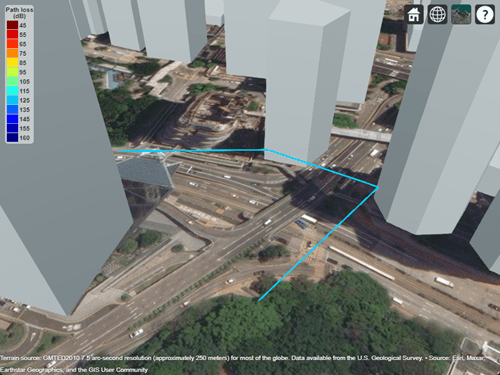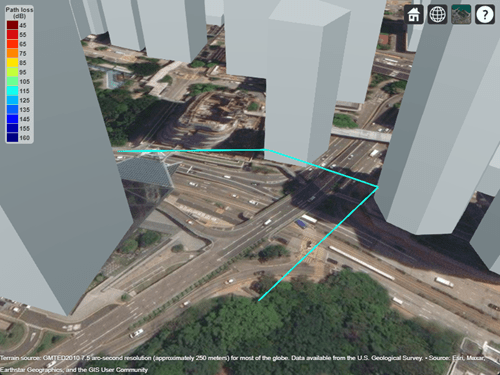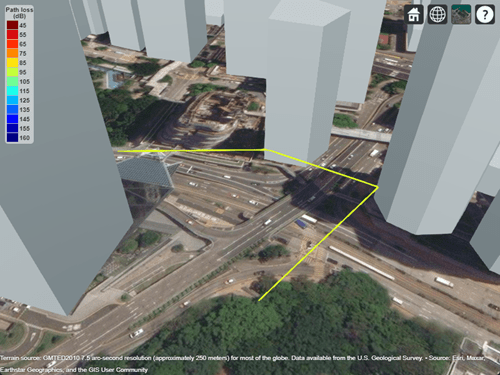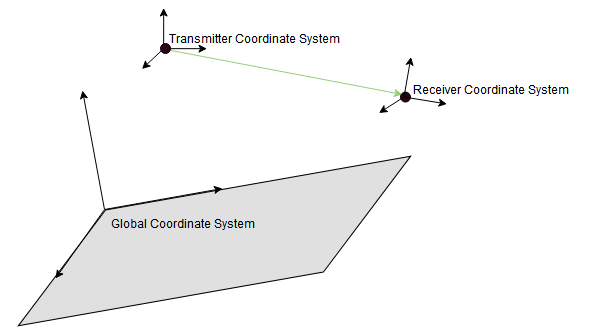# raypl

Path loss and phase change for RF propagation ray

## Syntax

``[pl,phase] = raypl(ray)``
``[pl,phase] = raypl(ray,Name,Value)``

## Description

example

````[pl,phase] = raypl(ray)` returns the path loss in dB and phase shift in radians based on the properties specified by `ray`. The path loss and path shift computations consider the free space loss and reflection loss derived from the propagation path, reflection materials, and polarizations. The function accounts for geometric coupling between horizontal and vertical polarizations only when both transmit and receive antennas are polarized. For more information, see Path Loss Computation.```

example

````[pl,phase] = raypl(ray,Name,Value)` calculates the path loss and phase shift with additional options specified by one or more name-value pair arguments.```

## Examples

collapse all

Change the reflection materials and frequency for a ray and reevaluate the path loss and phase shift.

```viewer = siteviewer("Buildings","hongkong.osm"); tx = txsite("Latitude",22.2789,"Longitude",114.1625, ... "AntennaHeight",10,"TransmitterPower",5, ... "TransmitterFrequency",28e9); rx = rxsite("Latitude",22.2799,"Longitude",114.1617, ... "AntennaHeight",1);```

Perform ray tracing between the sites.

```pm = propagationModel("raytracing", ... "Method","image", ... "MaxNumReflections",2); rays = raytrace(tx,rx,pm);```

Find the first ray with 2-order reflections from the result. Display the ray characteristics. Plot the ray to see the ray reflect off two buildings.

`ray = rays{1}(find([rays{1}.NumInteractions] == 2,1))`
```ray = Ray with properties: PathSpecification: 'Locations' CoordinateSystem: 'Geographic' TransmitterLocation: [3×1 double] ReceiverLocation: [3×1 double] LineOfSight: 0 Interactions: [1×2 struct] Frequency: 2.8000e+10 PathLossSource: 'Custom' PathLoss: 122.1824 PhaseShift: 4.5667 Read-only properties: PropagationDelay: 8.3060e-07 PropagationDistance: 249.0068 AngleOfDeparture: [2×1 double] AngleOfArrival: [2×1 double] NumInteractions: 2 ```
`plot(ray);`By default, all buildings have concrete building material electrical characteristics. Change the material to metal for the second reflection and re-evaluate path loss. Use the `raypl` function to reevaluate the pathloss for the ray. Display the ray path to compare the change in path loss. Replot to show the slight change in color due to the path loss change of the ray.

```[ray.PathLoss,ray.PhaseShift] = raypl(ray, ... "ReflectionMaterials",["concrete","metal"])```
```ray = Ray with properties: PathSpecification: 'Locations' CoordinateSystem: 'Geographic' TransmitterLocation: [3×1 double] ReceiverLocation: [3×1 double] LineOfSight: 0 Interactions: [1×2 struct] Frequency: 2.8000e+10 PathLossSource: 'Custom' PathLoss: 117.4814 PhaseShift: 4.5667 Read-only properties: PropagationDelay: 8.3060e-07 PropagationDistance: 249.0068 AngleOfDeparture: [2×1 double] AngleOfArrival: [2×1 double] NumInteractions: 2 ```
```ray = Ray with properties: PathSpecification: 'Locations' CoordinateSystem: 'Geographic' TransmitterLocation: [3×1 double] ReceiverLocation: [3×1 double] LineOfSight: 0 Interactions: [1×2 struct] Frequency: 2.8000e+10 PathLossSource: 'Custom' PathLoss: 117.4814 PhaseShift: 4.5667 Read-only properties: PropagationDelay: 8.3060e-07 PropagationDistance: 249.0068 AngleOfDeparture: [2×1 double] AngleOfArrival: [2×1 double] NumInteractions: 2 ```
`plot(ray);`Change the frequency and reevaluate the path loss and phase shift. Plot the ray again and observe the obvious color change.

```ray.Frequency = 2e9; [ray.PathLoss,ray.PhaseShift] = raypl(ray, ... "ReflectionMaterials",["concrete","metal"]); plot(ray);```Appendix

## Input Arguments

collapse all

Ray configuration, specified as one `comm.Ray` object. The object must have the `PathSpecification` property set to `"Locations"`.

Data Types: `comm.Ray`

### Name-Value Arguments

Specify optional comma-separated pairs of `Name,Value` arguments. `Name` is the argument name and `Value` is the corresponding value. `Name` must appear inside quotes. You can specify several name and value pair arguments in any order as `Name1,Value1,...,NameN,ValueN`.

Example: `raypl(ray,'TransmitterPolarization','H','ReceiverPolarization','H')`, specifies the horizontal polarizations for the transmit and receive antennas for `ray`.

Reflection materials for a non-line-of-sight (NLOS) ray, specified as a string scalar, 1-by-NR string vector, character vector, 1-by-NR cell array of character vectors, 2-by-1 numeric vector, or 2-by-NR numeric matrix. NR represents the number of reflections as specified by the `comm.Ray`.`NumReflections` property.

• When `ReflectionMaterials` is specified as a string scalar, string vector, or an equivalent character vector or cell array of character vectors, the reflection material must be one of `"concrete"`, `"brick"`, `"wood"`, `"glass"`, `"plasterboard"`, `"ceiling-board"`, `"chipboard"`, `"floorboard"`, `"metal"`, `"water"`, `"vegetation"`, `"loam"`, or `"perfect-reflector"`. When specified as a string scalar or char vector, the setting applies to all the reflections.

• When `ReflectionMaterials` is specified as a 2-by-1 numeric vector, the [relative permittivity; conductivity] value pair applies to all the reflections.

• When `ReflectionMaterials` is specified as a 2-by-NR numeric matrix, the [relative permittivity; conductivity] value pair in each column applies for each of the NR reflection points, respectively.

For more information, see ITU Permittivity and Conductivity Values for Common Materials (Communications Toolbox).

Example: `"ReflectionMaterials",["concrete","water"]`, specifies that a ray with two reflections will use electrical characteristics of concrete at the first reflection point and water at the second reflection point.

Data Types: `string` | `char` | `double`

Transmit antenna polarization type, specified as `"none"`, `"H"`, `"V"`, `"RHCP"`, `"LHCP"`, or a normalized [H; V] Jones vector. For more information, see Jones Vector Notation.

Example: `'TransmitterPolarization','RHCP'`, specifies right-hand circular polarization for the transmit antenna.

Data Types: `double` | `char` | `string`

Receive antenna polarization type, specified as `"none"`, `"H"`, `"V"`, `"RHCP"`, `"LHCP"`, or a normalized [H; V] Jones vector. For more information, see Jones Vector Notation.

Example: `'ReceiverPolarization',[1;0]`, specifies horizontal polarization for the receive antenna by using Jones vector notation.

Data Types: `double` | `char` | `string`

Orientation of the transmit antenna axes, specified as a 3-by-3 unitary matrix indicating the rotation from the transmitter local coordinate system (LCS) into the global coordinate system (GCS). When the `CoordinateSystem` property of the `comm.Ray` is set to `"Geographic"`, the GCS orientation is the local East-North-Up (ENU) coordinate system at transmitter. For more information, see Coordinate System Orientation.

Example: `'TransmitterAxes',eye(3)`, specifies that the local coordinate system for the transmitter axes is aligned with the global coordinate system. This is the default orientation.

Data Types: `double`

Orientation of the receive antenna axes, specified as a 3-by-3 unitary matrix indicating the rotation from the receiver local coordinate system (LCS) into the global coordinate system (GCS). The GCS orientation is the local East-North-Up (ENU) coordinate system at receiver when the .`CoordinateSystem` property of the `comm.Ray` is set to `"Geographic"`. For more information, see Coordinate System Orientation.

Example: `'ReceiverAxes',[0 -1 0; 1 0 0; 0 0 1]`, specifies a 90° rotation around the z-axis of the local receiver coordinate system with respect to the global coordinate system.

Data Types: `double`

## Output Arguments

collapse all

Path loss in dB, returns the path loss calculated for the input ray object, accounting for any modifications specified by `Name,Value` pairs.

Phase shift in radians, returns the phase shift calculated for the input ray object, accounting for any modifications specified by `Name,Value` pairs.

collapse all

### ITU Permittivity and Conductivity Values for Common Materials

ITU-R P.2040-1  (Communications Toolbox) and ITU-R P.527-5  (Communications Toolbox) present methods, equations, and values used to calculate real relative permittivity, conductivity, and complex relative permittivity for the common materials.

### Coordinate System Orientation

This image shows the orientation of the electromagnetic fields in the global coordinate system (GCS) and the local coordinate systems of the transmitter and receiver.When the `CoordinateSystem` property of the `comm.Ray` is set to `"Geographic"`, the GCS orientation is the local East-North-Up (ENU) coordinate system at observer. The path loss computation accounts for the round-earth differences between ENU coordinates at the transmitter and receiver.

### Path Loss Computation

The path loss computations in raypl follow the path loss and reflection matrix computations as described in IEEE Document 802.11-09/0334r8 . The function accounts for geometric coupling between horizontal and vertical polarizations only when both transmit and receive antennas are polarized.

For a first order signal reflection, the reflection matrix, Href1, is computed as

`${H}_{ref1}=\left[\begin{array}{cc}\mathrm{cos}\left({\psi }_{rx}\right)& \mathrm{sin}\left({\psi }_{rx}\right)\\ -\mathrm{sin}\left({\psi }_{rx}\right)& \mathrm{cos}\left({\psi }_{rx}\right)\end{array}\right]×\left[\begin{array}{cc}{R}_{\perp }\left({\alpha }_{inc}\right)& 0\\ 0& {R}_{\parallel }\left({\alpha }_{inc}\right)\end{array}\right]×\left[\begin{array}{cc}\mathrm{cos}\left({\psi }_{tx}\right)& \mathrm{sin}\left({\psi }_{tx}\right)\\ -\mathrm{sin}\left({\psi }_{tx}\right)& \mathrm{cos}\left({\psi }_{tx}\right)\end{array}\right]$`

The terms in the channel propagation matrix computation represent

• RX geometric coupling matrix — Recalculation of the polarization vector from the plane of incidence basis to RX coordinates.

• Polarization matrix — Matrix includes the reflection coefficients R⟂ and R∥ for the perpendicular and parallel components of the electric field E ⟂ and E ∥ respectively.

• TX geometric coupling matrix — Recalculation of the polarization vector from the TX coordinates basis to the plane of incidence.

This figure illustrates a first order reflected signal path.Where

• The reflection plane is offset from the global coordinate system origin.

• k represents the waveform propagation vector.

• n represents the vector normal to the incident plane.

• Eθ and Eφ represent the vertical and horizontal electromagnetic field vectors.

• αinc represents the incident angle of k.

• ψtx represents the angle between Eθ and a normal to the incident plane.

• TX represents the transmit antenna.

• RX represents the receive antenna.

The reflection matrix computations for second order signal reflections extend from the first order signal reflection computations. For more information, see IEEE Document 802.11-09/0334r8 .

### Jones Vector Notation

For Jones vector notation, the raypl function describes signal polarization using Jones calculus.

The orthogonal components of Jones vectors are defined for Eθ and Eφ. This table shows the Jones vector corresponding to various antenna polarizations.

Antenna Polarization TypeCorresponding Jones Vector

Linear polarized in the θ direction

`$\left(\begin{array}{c}H\\ V\end{array}\right)=\left(\begin{array}{c}0\\ 1\end{array}\right)$`

Linear polarized in the φ direction

`$\left(\begin{array}{c}H\\ V\end{array}\right)=\left(\begin{array}{c}1\\ 0\end{array}\right)$`

Left-hand circular polarized (LHCP)

`$\left(\begin{array}{c}H\\ V\end{array}\right)=\frac{1}{\sqrt{2}}\left(\begin{array}{c}j\\ 1\end{array}\right)$`

Right-hand circular polarized (RHCP)

`$\left(\begin{array}{c}H\\ V\end{array}\right)=\frac{1}{\sqrt{2}}\left(\begin{array}{c}-j\\ 1\end{array}\right)$`

 Maltsev, A., et al. "Channel models for 60 GHz WLAN systems." IEEE Document 802.11-09/0334r8, May 2010.

 International Telecommunications Union Radiocommunication Sector. Effects of building materials and structures on radiowave propagation above about 100MHz. Recommendation P.2040-1. ITU-R, approved July 29, 2015. https://www.itu.int/rec/R-REC-P.2040-1-201507-I/en.

 International Telecommunications Union Radiocommunication Sector. Attenuation by atmospheric gases. Recommendation P.676-11. ITU-R, approved September 30, 2016. https://www.itu.int/rec/R-REC-P.676-11-201609-S/en.